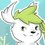# IMO 2016 Day 1

I'm surprised nobody else has posted this. Yes, there are plenty of discussions in Art of Problem Solving, but why not here? And of course there are more problems.

## Problem 1

Triangle $BCF$ has a right angle at $B$. Let $A$ be the point on line $CF$ such that $FA = FB$ and $F$ lies between $A$ and $C$. Point $D$ is chosen so that $DA = DC$ and $AC$ is the bisector of $\angle{DAB}$. Point $E$ is chosen so that $EA = ED$ and $AD$ is the bisector of $\angle{EAC}$. Let $M$ be the midpoint of $CF$. Let $X$ be the point such that $AMXE$ is a parallelogram. Prove that $BD$, $FX$, and $ME$ are concurrent.

## Problem 2

Find all positive integers $n$ for which each cell of an $n \times n$ table can be filled with one of the letters I, M, and O in such a way that:

• in each row and each column, one third of the entries are I, one third are M, and one third are O; and
• in any diagonal, if the number of entries on the diagonal is a multiple of three, then one third of the entries are I, one third are M, and one third are O.

Note. The rows and columns of an $n \times n$ table are each labelled $1$ to $n$ in a natural order. Thus each cell corresponds to a pair of positive integer $(i, j)$ with $1 \le i,j \le n$. For $n > 1$, the table has $4n-2$ diagonals of two types. A diagonal of first type consists all cells $(i, j)$ for which $i+j$ is a constant, and the diagonal of this second type consists all cells $(i, j)$ for which $i-j$ is constant.

## Problem 3

Let $P = A_1 A_2 \cdots A_k$ be a convex polygon in the plane. The vertices $A_1, A_2, \ldots, A_k$ have integral coordinates and lie on a circle. Let $S$ be the area of $P$. An odd positive integer $n$ is given such that the squares of the side lengths of $P$ are integers divisible by $n$. Prove that $2S$ is an integer divisible by $n$.Note by Ivan Koswara
4 years, 11 months ago

This discussion board is a place to discuss our Daily Challenges and the math and science related to those challenges. Explanations are more than just a solution — they should explain the steps and thinking strategies that you used to obtain the solution. Comments should further the discussion of math and science.

When posting on Brilliant:

• Use the emojis to react to an explanation, whether you're congratulating a job well done , or just really confused .
• Ask specific questions about the challenge or the steps in somebody's explanation. Well-posed questions can add a lot to the discussion, but posting "I don't understand!" doesn't help anyone.
• Try to contribute something new to the discussion, whether it is an extension, generalization or other idea related to the challenge.
• Stay on topic — we're all here to learn more about math and science, not to hear about your favorite get-rich-quick scheme or current world events.

MarkdownAppears as
*italics* or _italics_ italics
**bold** or __bold__ bold
- bulleted- list
• bulleted
• list
1. numbered2. list
1. numbered
2. list
Note: you must add a full line of space before and after lists for them to show up correctly
paragraph 1paragraph 2

paragraph 1

paragraph 2

[example link](https://brilliant.org)example link
> This is a quote
This is a quote
    # I indented these lines
# 4 spaces, and now they show
# up as a code block.

print "hello world"
# I indented these lines
# 4 spaces, and now they show
# up as a code block.

print "hello world"
MathAppears as
Remember to wrap math in $$ ... $$ or $ ... $ to ensure proper formatting.
2 \times 3 $2 \times 3$
2^{34} $2^{34}$
a_{i-1} $a_{i-1}$
\frac{2}{3} $\frac{2}{3}$
\sqrt{2} $\sqrt{2}$
\sum_{i=1}^3 $\sum_{i=1}^3$
\sin \theta $\sin \theta$
\boxed{123} $\boxed{123}$

## Comments

Sort by:

Top Newest

I have an extension to Q1. (Almost got a complete solution for it, but i observed this, which follows):

Let this intersection point be labelled $O$. Prove $O$ is the circumcentre of $\Delta XBE$.

- 4 years, 11 months ago

Log in to reply

Nice observation. If you complex trig bash it, the solution to your extension becomes very apparent. I'm currently typing up my solution to the original problem and can include your extension at the end.

- 4 years, 11 months ago

Log in to reply

Nice, I have my own solution for it as well. Th essence for this extension is to just prove $OE=OX$ since $OX=OB$ is quite apparent.

- 4 years, 11 months ago

Log in to reply

Here's my synthetic solution. Let $XF$ intersect $BD$ at $O$ and $EM$ at $O^{'}$. We need to show that $O$ coincides with $O^{'}$. A clear observation shows that $B,C,F,D,X$ are concyclic because $\angle\{DCF\}$=$\angle\{DBF\}$. Moreover $D$ is the circumcenter of triangle $CBA$. A clear angle chase shows that $\angle \{DXF\}$=$\angle\{DBF\}$ showing $O$ as the circumcenter of triangle $OBE$ thus $E{O^{'}}$=$BO$ Thus $O$ coincides with $O{'}$.proved Your extension can be proved easily if you watch $F$ as the incenter of triangle $DAB$ and watching $XFAD$ and $CDEM$ as the parallelogram. Thanks proving $O$ as the circumcenter was really very helpful.

- 3 years, 3 months ago

Log in to reply

Oh about it, u were talking to me.. OK I am also trying to solve it with another way...

- 3 years, 3 months ago

Log in to reply

Provide a solution using complex numbers.

- 3 years, 3 months ago

Log in to reply

Well ur solution was really nice, I am also seeing it and trying to find a mistake..... 😂 😂😂😂😂😂😂😂😇😇😇😇😇😝😝😝😝😝😋

- 3 years, 3 months ago

Log in to reply

×

Problem Loading...

Note Loading...

Set Loading...